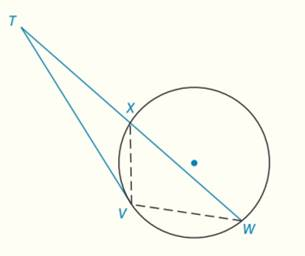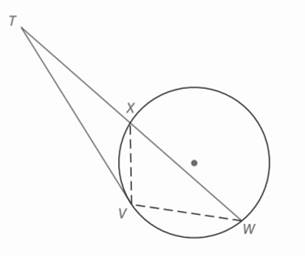Chapter 6.CT, Problem 12CT### Elementary Geometry for College St...

6th Edition
Daniel C. Alexander + 1 other
ISBN: 9781285195698

#### Solutions

Chapter
Section### Elementary Geometry for College St...

6th Edition
Daniel C. Alexander + 1 other
ISBN: 9781285195698
Textbook Problem
1 views

# In the figure, T V ¯ is a tangent and Δ T V W ∼   Δ T X V . Find T V if T X = 3 and X W = 5 .                                   _To determine

To find:

TV from,.

Explanation

Given:

TV¯ is a tangent and ΔTVWΔTXV.

TX=3 and XW=5.

Theorem used:

“ If a tangent segment and a secant segment are drawn to a circle from an external point, then the square of the length of the tangent equals the product of the length of the secant with the length of its external segment”.

Calculation:

Consider,

From the theorem, “ If a tangent segment and a secant segment are drawn to a circle from an external point, then the square of the length of the tangent equals the product of the length of the secant with the length of its external segment” we can have,

TV2=TXTW(1).

Here,

### Still sussing out bartleby?

Check out a sample textbook solution.

See a sample solution

#### The Solution to Your Study Problems

Bartleby provides explanations to thousands of textbook problems written by our experts, many with advanced degrees!

Get Started

#### In Exercises 33-38, rewrite the expression using positive exponents only. 38. (x y)(x1 + y1)

Applied Calculus for the Managerial, Life, and Social Sciences: A Brief Approach

#### What is the lowest score in the following distribution?

Essentials of Statistics for The Behavioral Sciences (MindTap Course List)

#### Calculate y'. 25. sin(xy) = x2 y

Single Variable Calculus: Early Transcendentals, Volume I

#### Define fraud and explain the safeguards that exist to prevent it.

Research Methods for the Behavioral Sciences (MindTap Course List)